# Ore Reserve Estimation Method

Estimates of the tonnage and average grade of ore deposits (ore-reserve estimates) are made for various purposes. They may be made by examining engineers as a basis for placing a value on a mining property in connection with reports on behalf of owners or vendors, on the one hand, or for prospective purchasers or lessees, on the other. They are made for tax purposes and sometimes in connection with mergers of two or more companies or with litigation.

Most operating companies make periodical ore-reserve estimates, usually at least annually, to determine their ore-reserve position as a basis for controlling development and exploration and allocation of funds therefor; for determining deferred, depletion, and depreciation charges per ton; or as a basis for deciding upon operating policy—expansion or contraction of operations, capital expenditures, and the like.

The final estimate in any instance usually is a composite of estimates of different blocks or areas which often differ appreciably from each other in grade or character of ore. A continuous ore-reserve inventory, by blocks, levels, and stopes, in a mine may be required as a basis for controlling stoping operations to maintain a desired grade of output by mixing ores from different blocks.

Some mines produce two or more grades or kinds of ore, which must be mined, milled, and shipped separately; and it becomes necessary, then, to know the grade and type of ore available in each section of the mine.

Thus, iron ores are often mixed to give a desired iron, phosphorus, silica, manganese content. The copper-bearing sulfide ores of Ducktown, Tenn., chiefly valuable for their sulfur, are roasted for the manufacture of sulfuric acid; it is necessary to grade the ore from different stopes carefully, on the basis of sulfur content, to form the right mix for this purpose.

Ore-reserve estimates are based upon the results of exploration and development and analyses of the samples derived therefrom. Unless a deposit is fully developed (and even then to a lesser degree), certain assumptions have to be made regarding the continuity and grade of ore between exposed faces or drill holes that have been sampled. In making these assumptions, the engineer must interpret all available information, and the accuracy of the final results will depend largely on his experience and the soundness of his judgment. Not only must he correctly combine the assay values of the samples, but he must, interpret the geological criteria and consider the influence of structural conditions on continuity and grade of ore, the probable loss of ore in mining, the dilution with waste (which, in turn, may depend on the mining method employed or to be employed), and the cost of mining and milling, which may be an important factor in determining the minimum grade of rock that can be classed as ore.

It is apparent, then, that estimation of tonnage and grade of ore reserves is not a precise science. In some districts where the character, uniformity, and habit of the ore bodies have been learned from long mining experience, more or less arbitrary methods may be applied with considerable confidence in estimating ore extending beyond exposed faces. Thus, in Minnesota and Michigan the mining companies and the State tax commissions accept valuations of ore reserves based upon certain rules as to continuity and grade of the ore.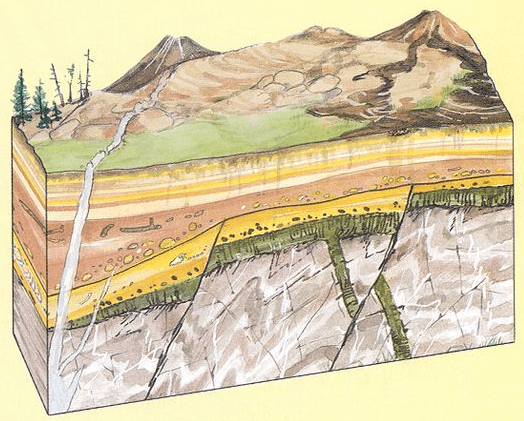Ore-reserve estimates include the determination of (1) tonnages of ore and (2) average grade or value per ton. Since the grade or content of valuable metal establishes the difference between rock that may and may not be classed as ore, tonnage cannot be estimated without considering the question of grade.

Consideration of grade in connection with tonnage estimates may or may not present a complex problem. Thus, in some deposits with sharply defined walls or boundaries between which all the material is known by experience or by actual development to be of ore grade without the necessity of averaging the sample assays, the tonnage can be estimated without particular reference to assays. In other types of ore bodies having no definite boundaries and with values gradually petering out, the limits of minable ore have to be determined by assay; hence, tonnage and grade estimates are interdependent. With large low-grade deposits that can be mined profitably only by use of large-scale non selective methods, it may be necessary to compute tonnages and grades on the basis of several different assumptions as to the mining limits. The first estimate may indicate that too much marginal ore has been included to give an average grade that will leave the required profit margin, and it then becomes necessary to establish new hypothetical ore boundaries and re-estimate the average grade of the corresponding tonnage.

This process may require repetition by cut-and-try methods before a final acceptable estimate is reached. It involves a determination of the minimum grade of material that can be included, but the mining method to be employed may preclude the possibility of eliminating all material below this grade, hence the necessity for cut-and-try estimates in such instances.

Some deposits are so uniform in grade that satisfactory ore-reserve estimates can be made by merely computing the volume and tonnage of ore and assigning to it the grade of the ore produced during the preceding year or other period. Except for such deposits, the initial step in making ore-reserve estimates is the preparation of assay maps and sections. These not only furnish the data necessary for actual measurements and computations, but provide a general picture of the ore bodies, the variations in grade within them and along their boundaries, and thus the nature of the irregularities of mineralization.

In making ore-reserve estimates as a basis for valuing a property for sale or other purpose, most engineers, to avoid overvaluation and at the same time recognize potentialities for ore as yet undisclosed, estimate tonnages under three separate headings: “Developed ore” (sometimes termed “positive ore”), “probable ore,” and “possible ore.” The judgment of the engineer must be relied upon to a considerable extent in drawing the distinctions between these different classes of ore. In general, however, developed ore is restricted to ore already broken in the stopes plus ore exposed on four sides—that is, ore that actually can be measured. Of course, if the exposed faces of a block of ore are far apart, an assumption is necessary regarding the continuity of ore between them, and the accuracy of the result again depends on the estimator’s judgment.

In estimating probable ore, somewhat more latitude is allowed. It is usual, however, to class as probable ore only that exposed and measurable on at least two sides, or measurable on one side and exposed at one point on the opposite side of a block. In the latter instance it is customary to compute a tonnage based on the area of a triangle having as its base the exposed side and its apex the exposed point on the opposite side. In the probable ore tonnage, there also may be included some ore extending beyond exposed faces, provided known geological conditions warrant the expectation that the ore will continue a given conservative distance below a level or beyond a drift face.

In estimating possible ore, still more latitude may be permissible. The estimator will be influenced by past experience as to extensions of ore if the mine is an old one and by geological conditions known to affect localization of ore shoots.

Since the greatest present value of a mining property actually may lie in its potential ore (probable and possible ore) rather than in its developed ore, estimates of the former may be of utmost importance. This value can hardly be computed by formulas or by any fixed rules, and the judgment of the estimator, his experience, knowledge, and integrity comprise the only measure of the reliance that can be placed on his appraisal. In any event, since the estimated tonnages and grades of probable and possible ore are more subject to error than are those of fully developed ore, it is logical to set up ore reserves under three classes to indicate the degree of reliance that can be placed on each.

## Assay Maps and Sections

Assay plans may be prepared in various ways, depending on the types of the ore bodies. Whatever the method employed, the position of each sample, its length, and its assay value should be posted on the maps or sections. If the plans are to be submitted as part of a report that is to be or may be checked by another estimator, it is important that they be posted in this detail. Engineers often are called upon to check reports in which these details are omitted and only the results of computations of the original estimator are given. In some instances the figures merely show the computed tonnage and average grade of each of several blocks of ore. In others, the computed average assay values of each of the sides of the ore blocks or averages of groups of samples covering given lengths of ore exposures are shown, as, for example, “50 feet, 10 samples, average value \$9.50, average width 6.5 feet.” In such instances, the calculations cannot be checked in their entirety and the check estimator is left in the dark on such points as the treatment that has been accorded erratic high assays. As will be shown later, one erratic high assay averaged with several assays well below average ore grade may give an erroneous result considerably above the actual grade. Assay plans may consist of plan maps of the workings, drill holes, and ore bodies, vertical cross sections and longitudinal sections, or projections on the planes of the veins, or combinations of these.

## Volume and Tonnage Estimates

The plans are drawn to scale, and from them areas may be scaled or measured with a planimeter. Areas are commonly figured in square feet and ore volumes in cubic feet (except where the metric system is employed). Volumes in cubic feet are then converted into tons by dividing by the number of cubic feet in a ton of the rock or ore in place as determined by specific gravity or other tests.

## Ore Volume Calculation

For flat-lying, bedded deposits of uniform thickness, volume computations involve merely scaling or measuring with a planimeter the areas of the different blocks of ore bounded by mine workings and the margins of the ore bodies and multiplying the area of each block by the average thickness of the ore in that block. Summation of the volumes of all blocks gives the total volume of the ore.

For broad, basin-shaped deposits, plan maps, vertical or horizontal cross sections or both may be used. If the deposit has been explored by vertical drill holes or test pits, a common method is to plot the holes in plan and divide the area into a series of triangles, squares, or irregular polygons, each of which represents the horizontal cross section of an imaginary vertical prism of ore extending from the top to the bottom of the deposit. The volume of the prism is the area of the cross section multiplied by the depth, if the top and bottom of the prism are plane parallel surfaces. Since in deposits of this type the top and bottom may be very uneven, this method is an approximation; the closer the spacing of the holes, and thus the greater the number of prisms, the more closely will the approximation approach the actual volume. The sum of the volumes of all the prisms is then the total volume of ore in the deposit.

Sometimes holes are drilled at regular intervals at the apexes of equilateral triangles or at the corners of equal squares. More often, on account of topography or irregularities of the deposit, this is not possible and the arrangement is very irregular. The areas of influence or “weights” of the different holes then vary, sometimes considerably, with respect to both volume and ore-grade calculations. Complicated methods for constructing geometric figures to determine these factors have been described and discussed in textbooks and the technical press. However, competent mathematicians consulted by the authors agree that none of them is mathematically correct for all drill-hole arrangements and variations in grade. On the other hand, simple constructions are possible from which results may be obtained that are well within the order of accuracy of other factors entering into ore-reserve calculations; for example, errors in sampling and in assumptions as to ore boundaries may exceed the errors in approximations derived from these simpler methods.

Since the method of constructing these figures also affects the calculations of ore grade, this subject will be discussed further under the caption, Estimation of Grade of Ore.

The volume of large basin-shaped deposits may also be estimated from parallel cross sections on which are plotted intersections or projections of mine workings, drill holes, and ore boundaries. It is often convenient to draw vertical cross sections through lines of drill holes, raises, and winzes, for which purpose it may be necessary to prepare longitudinal sections and plans, or bottom- and top-of-ore contours from which points on the cross sections may be derived by interpolation. Having prepared a series of parallel cross sections, the area of each is computed or is measured by planimeter. If the variation in size and shape of adjoining sections is small, the volume in cubic feet of the block between them may be taken, for all practical purposes, as their average area multiplied by the distance between them in feet. If there is a series of such sections spaced equidistantly, the total volume may be expressed by the “end-area” formula:

V = ½ H (A1 + 2A2 + 2A3 + An)

where A1, A2, A3 An are the areas of the several sections,
H is the perpendicular distance between them,
and V is the total volume.

If the ore body is very irregular and adjoining sections exhibit marked dissimilarity in size and shape, the volume between two adjoining sections may be approximated more closely by using the prismoidal formula:

V = H/6 (A1 + 4M + A2).

where V = volume of the block,
H is the perpendicular distance between A1 and A2, A1 and A2 are the areas of the two sections,
and M is the area of a parallel section halfway between A1 and A2.

M is determined by constructing a midsection interpolated from corners of sections A1 and A2, modified by any information available concerning outlines between A1 and A2 that may be obtained from mine workings or geological data.

Figure 10 shows two adjacent sections that are quite dissimilar in shape. By using the end formula, the volume is 17,737,500 cubic feet, but by using the prismoidal formula it is 18,162,500, a difference of 425,000 cubic feet, or 2.5 percent.

If enough data are available to construct sections at close intervals, the prismoidal formula may be used, making each alternate section a midsection.

For dipping veins or lenses and other tabular forms of deposits, estimates are made from level plans, cross sections, and longitudinal projections on the plane of the dip, drawn to scale. All the assay widths and values are shown on these drawings, together with mine workings and outlines of blocks of ore remaining as determined by development or assumed from geological and other controlling data. The areas of the different blocks of ore multiplied by the corresponding average thickness of each, as determined from measurements normal to the dip, give the volumes of the respective blocks.

## Ore Tonnage Calculations

Tonnage estimates are derived from those of volume by applying a specific gravity or “tonnage” factor. The specific gravity of the mineral mass may be calculated roughly from the specific gravity and percentage of each of the constituent minerals and the porosity of the ore, or by making direct specific-gravity tests on representative samples from various parts of the ore body. Cubic feet per ton is then obtained by dividing 2,000 (2,240, if long tons are desired) by the product of 62.5 (weight per cubic foot of water) multiplied by the specific gravity of the ore (2,000/62.5 x sp. gr.). A more accurate tonnage factor may be determined by actually weighing the ore from measured volumes of excavation and averaging the results. A precise factor cannot, as a rule, be obtained for most large ore bodies because of the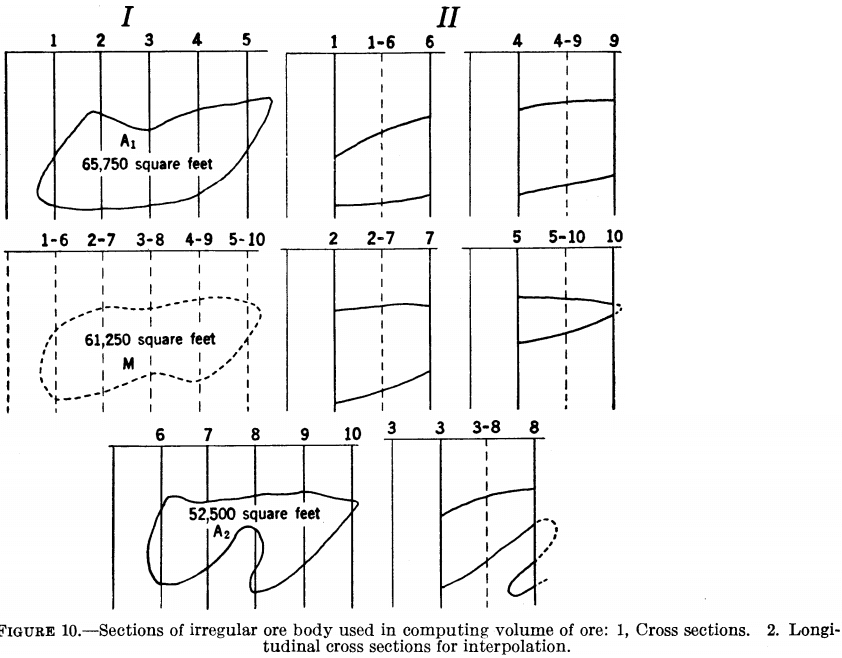variations in mineral composition and in porosity from point to point, but a factor accurate enough for all practical purposes usually can be derived by the methods described.

In some instances there may be enough variation in the specific gravity of different grades of ore to require different factors for each. Thus, in the Lake Superior iron-ore districts curves have been constructed showing the variation in cubic feet per ton with variations in iron content. One of these is shown in figure 11, A. At Cananea, Mexico, the specific gravity has been found to vary considerably with the copper content (fig. 11, B).

When these variations are substantial and are approximately known, it may be advisable, first, to calculate from the assay plans the average grade of each block of ore and then calculate the tonnage by employing the tonnage factor applicable to each grade. The tonnage in each block of ore is, of course, the volume in cubic feet divided by the cubic-feet-per-ton factor (or similar calculations for metric units).

When it comes to actual mining, it often happens that the tonnage is greater and the grade of ore lower than estimated. This often is due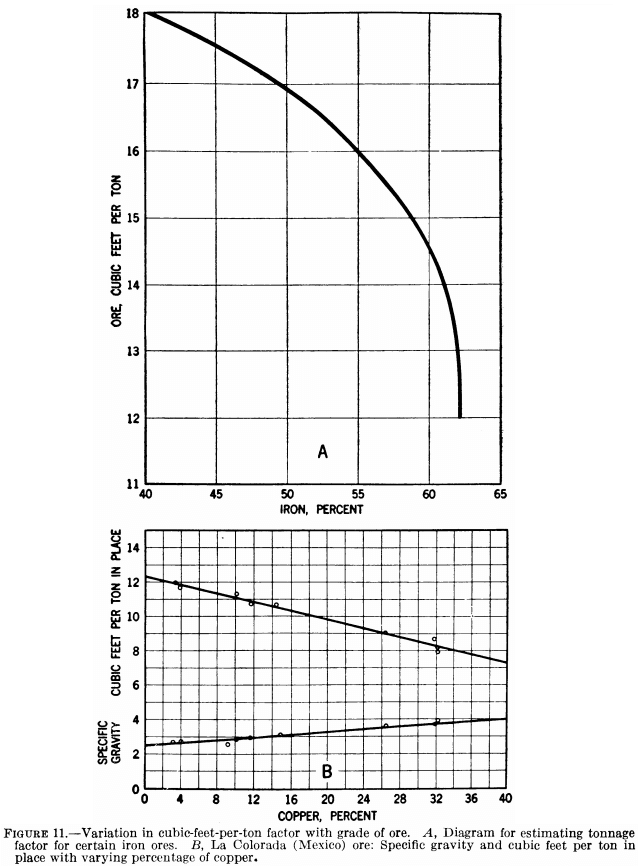largely to dilution from the walls in stoping, though it may be due partly to mining of marginal ore of lower grade than that included in the estimates. There may be compensating factors, such as offshoots of high-grade ore not known to exist at the time of the estimate or unexpected inclusions of waste within the ore bodies. Allowance usually is made for possible or probable inclusion of waste and loss of ore in mining, by deducting a certain percentage from the calculated tonnage. Probable dilution from the walls may be compensated for by adding a certain percentage to the tonnage estimate and correspondingly reducing the calculated grade of ore. These percentages may be arbitrary or may be based upon experience with deposits of the particular type in question and with the mining method to be used. Thus, in one district experience has shown that a 10-percent deduction should be made for loss of ore in mining and another 10 percent for inclusion of waste. In another district it is necessary to allow for 20 percent dilution from the walls during mining; that is, the calculated tonnage must be divided by 0.80 and a corresponding reduction made in the calculated grade of ore.

As previously indicated, the accuracy of ore-grade estimates will depend on the accuracy of the samples, accuracy in assaying, the method of combining and weighting the different samples, and the estimator’s judgment.

It has been shown that individual samples may be relatively inaccurate, but the weighted average of a large number may closely approximate the actual grade of the ore. Accuracy in assaying may be achieved with care and correct laboratory technique. The proper combining and weighting of sample assays may require considerable thought, and correct mathematical principles should be employed, at least up to the point where the mathematical precision equals the accuracy of the data upon which the calculations are based.

## Estimates based on Drill-Hole Data

Sometimes it may be necessary, before combining and averaging the assay values of the different samples, to make some preliminary computations. This applies particularly where the samples have been obtained by core drills, if the core recovery is incomplete, or if there is an appreciable difference between core and sludge assays. In such instances it becomes necessary to average the assays of core and of sludge and in doing so to assign proper weight to the value of each. In weighting, certain assumptions must be made, and therefore the results will not be precise. If it is assumed that the hole and core are uniform in diameter over the length of the sample, that there have been no additions of material by caving in the upper portions of the hole, and that all of the cuttings (sludge) have been caught, simple arithmetical calculations can be made. In such case the cuttings will represent all that part of the material removed that is not core, and the weights assigned to core and sludge assays will be proportional to their respective masses. The relative masses have been calculated for standard sizes of diamond-drill bits and different lengths of core recovery from a 5-foot hole and tabulated. Table 10 has been prepared by the E. J. Longyear Co. It is seen that even with complete core recovery the mass of the cuttings is greater than that of the core when the smaller bits are used.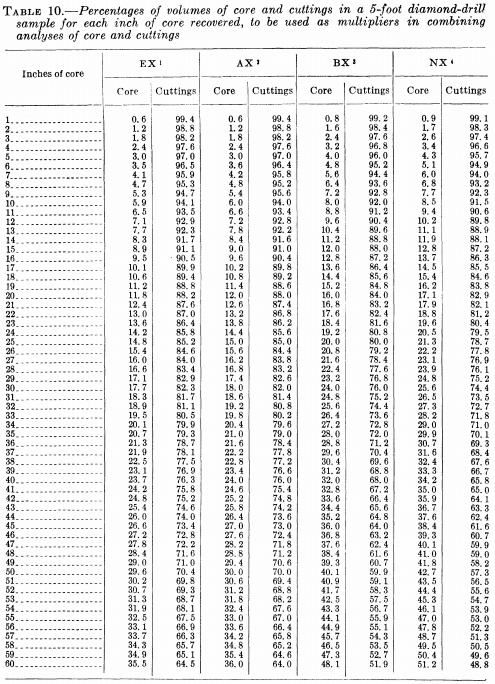When the core from a given section or “pull” is broken into a number of pieces it may not be possible to measure accurately the length of core recovered. As a check against the measurement, the core may be weighed, and this weight divided by the weight per inch of a core of the same diameter (calculated or determined from actual weighing) will give the equivalent length of core recovery.

The method of using the table may be illustrated by a hypothetical example. Assume a 5-foot section of EX diameter hole, 48 inches of core, a core assay of 3.0 percent copper, and a sludge assay of 3.6 percent copper. The core assay would receive a weight of 28.4 and that of the .sludge 71.6. The calculation then becomes:

Average assay = 1/100 (28.4 x 3.00 + 71.6 x 3.6) = 3.43 percent copper

If a portion of the cuttings is lost or other material is added from above a section of the hole under consideration, or if the hole is larger in diameter in softer portions of drill-hole section than in the harder sections, the weightings indicated by the table will not give correct average values when there is considerable difference between the assay-values of core and cuttings. Stated another way, and quoting from Longyear:

If a portion of the cuttings is lost or other material is added, the sample will be correct only to the extent that the material analyzed represents a true sample of the actual cuttings.

In actual practice complete sludge recovery, entire absence of salting by caving, and holes of uniform diameter probably are the exception rather than the rule. These conditions, however, may be approximated in many instances so that no serious error is introduced by employing the proportional-volume method of combining sludge and core assays.

When there is considerable deviation from these ideal conditions, the estimator must use his judgment in combining core and sludge assays. At some mines it is the practice to disregard the sludge entirely, in others to apply a factor to the assays of core or sludge, or a combination of both, this factor being based upon experience already gained with the particular ore in question.

A serious error may be introduced in some cases if sludge assays are ignored. For example, consider an ore composed of alternate hard and soft layers, in which the soft layers contain a considerably higher percentage of valuable mineral than do the hard layers. In this instance, the core usually will be of smaller diameter and the outside diameter of the hole will be larger in the soft layers than in the harder layers. There will then be too small a porportion of the high-grade material in the core and too large a proportion in the sludge.

At Morenci, Ariz., the practice was to calculate the average copper content by combining the assays of core and sludge in proportion to their respective weights. If the weight of the sample did not correspond with the calculated weight of the corresponding section of drill hole, the results were not given full weight and adjustments were made in the calculated grades.

In Northern Rhodesia a method of combining core and sludge assays based upon the following formula was developed:

Percent copper in core and sludge equals (weight of total core times percentage of copper in combined core plus weight of sludge times percentage of copper in sludge) divided by (weight of core plus weight of sludge).

Having prepared the necessary plans and cross sections and placed thereon the assays of the drill-hole samples, Which may have to be computed in some instances as outlined above, the next step is the combining of assays to obtain the average grade of different blocks or layers of ore and finally the average grade of the calculable ore reserves.

There are two general types of deposits of large area prospected by vertical drill holes: (1) Massive, irregular, basin-shaped, or flat-lying deposits with irregular or uniform mineralization, and (2) basin-shaped deposits in which different grades of ore are present in distinct layers.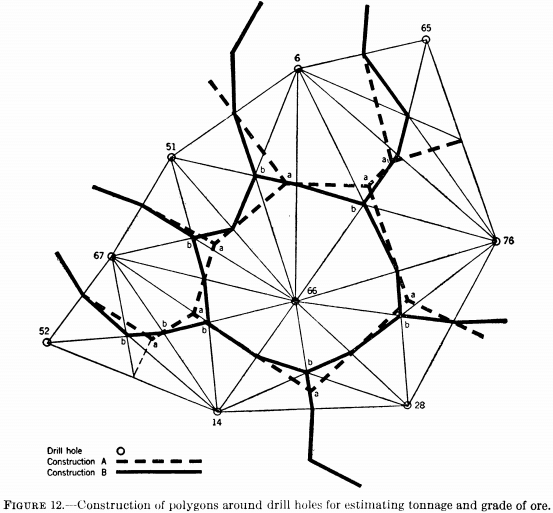## Ore Reserve Estimate EXAMPLE 1

In the first case, the triangular or polygonal prism method may be employed for computing tonnages and grades of ore. With this method each prism of ore is assumed to have the average grade of the drill hole around which it is constructed, and the geometrical construction should be such that this assumption will produce a result as nearly in accordance with the actual facts as possible.

There are two general methods of construction based on somewhat different hypotheses, viz: A. “That between any two sampling columns, the grade of ore of either one is assumed to extend halfway, and halfway only, to the other; and that in any prism, the grade of ore at any point is assumed to be that of the nearest assayed sample.” B. That variations in grade from one assayed sample point to the next are “straight-line” variations; that the grade at any point in a plane through three assayed sample points is influenced by the grade of all three in inverse ratio to the distance from each.

The constructions based on these two hypotheses are also different and produce different results, as indicated by figure 12. With either construction, however, the procedure is mechanical and eliminates the personal equation in computation, and two different estimators using the same method will arrive at the same answer. Figure 12 shows in plan a number of drill holes forming part of a larger drill-hole pattern. The broken lines show the construction and resulting polygon around hole 66, based on hypothesis A, whereas the solid lines show the polygon resulting from construction B.

In both constructions, the first step is to draw lines joining each hole to every other contiguous hole to form a series of triangles. In construction A, perpendiculars are erected from the centers of each side of each triangle intersecting at a common point as “a-a . . . a.” By joining these points, a polygon is formed around each hole, which is assumed to represent the cross section of a prism of ore of the same grade as the hole. It may be noted that with this construction, a may fall entirely outside its triangle in certain cases, as at (obtuse triangles).

In construction B, a line is drawn from each apex of a triangle to the center of the opposite side, the three lines intersecting at a common point, b, in the center of the triangle and dividing it into three smaller equal triangles. If the grade at the center of the large triangle, as determined by relative distances from each apex, be multiplied by the area of triangle, the result will be the same as that obtained by multiplying the grade of each hole by one-third the area of the large triangle and adding the products. The broken heavy and solid heavy lines show the respective polygons and the light lines the constructions for A and B. The average grade of each hole is the sum of the products of each sample length by its assay value divided by the sum of the lengths. This grade, multiplied by the volume of the prism and divided by the volume-per-ton factor, gives the tons- percent or ton-dollars in the prism, as the case may be. Adding these products for all the prisms and dividing by the sum of the tonnages gives the computed average grade of the deposit.

## Ore Reserve Estimate EXAMPLE 2

In the second case, vertical cross sections of the deposit are best adapted to making the estimates. The drill holes are drawn on the sections, the samples and assay values are plotted, and points in adjoining holes having similar analyses indicating portions of the same ore layer are joined by lines, thus marking the tops and bottoms of the layers (fig. 13).

For any section, the average assay value of a layer is computed as follows: The average assay value of each hole for the layer in question is assigned to the area extending from the hole halfway to the holes on each side; the sum of the products of each area times the corresponding assay value, divided by the sum of the areas, gives the average assay of the layer on that section. The average assay value and area of the corresponding layer are calculated for all the cross sections in the same manner. The volume of the layer in the block between two sections is found by the end-area formula or by the prismoidal for-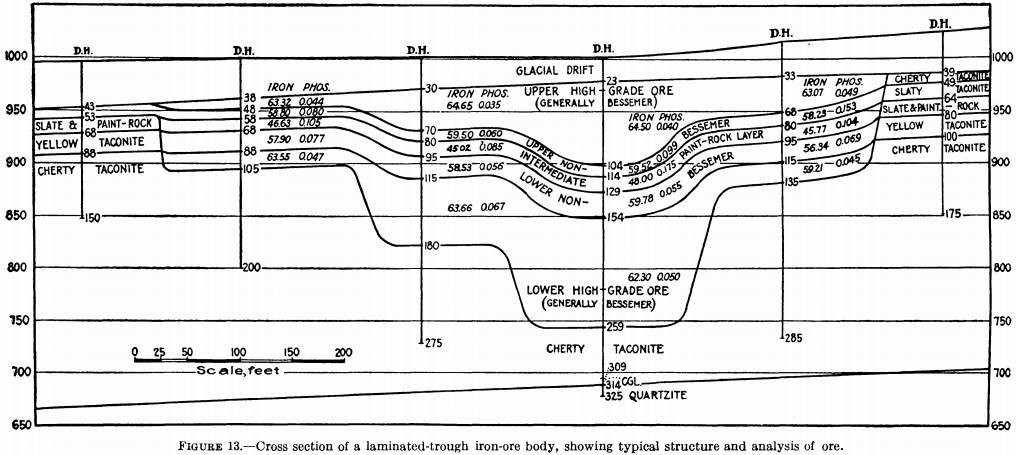mula, and this divided by the volume-per-ton factor gives the corresponding tonnage. The average assay value of this block is computed then from the formula V = (A1V1 + A2V2)/(A1 + A2) ; where V is the average value of the block, V1 and V2 are the values of the respective sections, and A1 and A2 are the corresponding section areas. Adding the products of the tonnages of the several blocks by their corresponding average assay values and dividing this sum by the sum of the tonnages gives the average assay value of the layer: V = V1T1 + V2T2 + VnTn/T1 + T2 + Tn where V is the average value of the layer, V1 Vn, the average value of the several blocks, and T1 Tn, the corresponding tonnages in the blocks. Values and tonnages of the other layers of ore are computed similarly.

## Estimates based on Underground Sampling

It has been pointed out that in sampling mine workings, the samples should be taken at regular intervals to avoid errors that might result from introduction of the human factor in the selection of sampling points. With a regular interval the calculations of ore grade are simplified because no weighting of assay values for ore length is required.

## Weighting of Assays of Individual Samples

Each sample, however, must be weighted in proportion to the length of the sample cut across the ore body. Thus, referring to figure 7, if samples 1 to 5 are, respectively, 1.0, 2.5, 3.0, 2.75, and 2.8 feet long and the assay values are, respectively, \$0.50, \$5, \$20, \$4, and \$25, the average grade of the face or section is computed as follows:

First compute the average grade of the band represented by samples 3 and 5.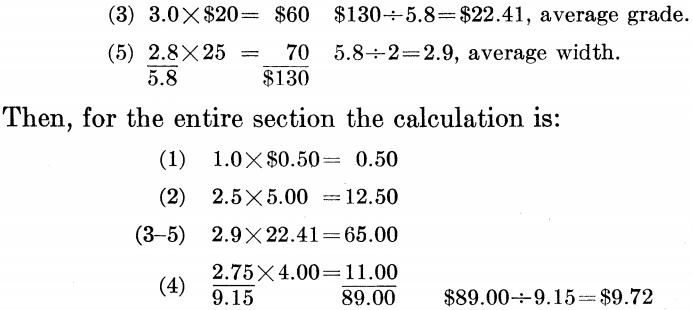Similarly, in averaging the assay values of a series of sample sections along the back of a drift, the sum of the products of the assay values of the several sections times the respective section widths divided by the sum of the section widths gives the average assay value of the series, provided the sections are equally spaced. If not equally spaced, the average may be computed by the formula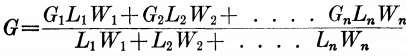where G is the average grade, G1, G2, etc., are the grades of the several sections, L1, L2, etc., the lengths of ore, and W1, W2, etc., the widths represented by the corresponding sections. G1, G2, etc., may be either assay values of single samples, where only one sample is taken for each section across the drift, or may be computed weighted averages where the drift section is composed of several samples representing different bands.

## Erratic High Assays

When posting the assays on the assay plans and sections, occasional so-called erratic high assays may be found that require special treatment before they are entered in the computations. Although recurrence of such assays indicates the probable presence of high-grade spots or streaks in the ore body, which, if they occur often enough, may actually raise the average grade of the ore body appreciably, experience has proved that, if taken at their full value, the resulting estimates will be too high. When it is considered that in the majority of instances the erratic highs represent a condition occurring at only the particular spot where the sample was cut and are much higher than the average grade of the section or block of ore they influence, the need for caution in evaluating them can readily be understood.

Therefore, although mathematically there may be no sound reason for eliminating or reducing the high assays, the conservative practice is to reduce them in accordance with some empirical rule or by re-averaging. Often check-sampling of a high section may be necessary before recalculating.

Empirical rules may be based upon experience with the ore in a particular deposit. Thus, at the McIntyre mine in the Porcupine district of Ontario erratic high assays among average low ones were arbitrarily cut to \$50; where they are consistently high they are not reduced.

At the Hollinger mine, in the same district, erratic high assays were cut to \$100 in stopes known to be high-grade and to \$50 in low-grade stopes. Where diamond-drill-hole assays are used in ore-reserve estimates, high assays are cut to \$10. Ore showings in isolated diamond-drill holes are not included in ore-reserve estimates. Where the drill-hole sections are between faces of developed ore, the assays may be included, but high assays are cut to \$10.

At these mines, the practices described have proved, over a period of years, to give accurate results.

Another practice employed at some mines is to average the erratic high assay with the assays of the two adjoining samples and insert this average in place of the erratic high in the final computation, as illustrated by the following example: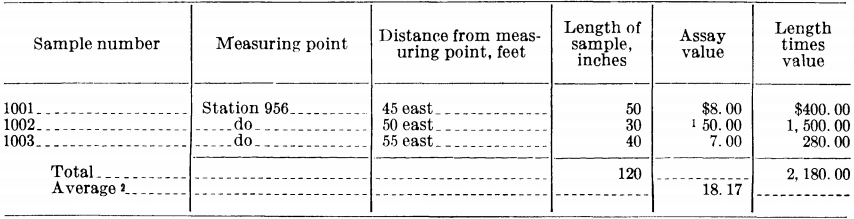The figure \$18.17 is then inserted in place of \$50 in the final computation.

A method virtually the same as the foregoing has been employed at the Wright-Hargreaves mine, Kirkland Lake, Ontario. In estimating the grade of a given block of ore or averaging the assays along a section of a drift, all the assays, including the erratic highs at their full value, are first averaged by the dollar-foot method. A second average is then made, the first average being inserted in place of each erratic high. Values estimated in this manner have checked actual bullion recovery plus tailing loss within 5 percent; in some months the estimates are high and in others low, so that over a period of a year or more the average error is less than 5 percent (plus or minus). Following is a sample calculation in which round figures were used for grades and lengths of samples for the sake of simplicity.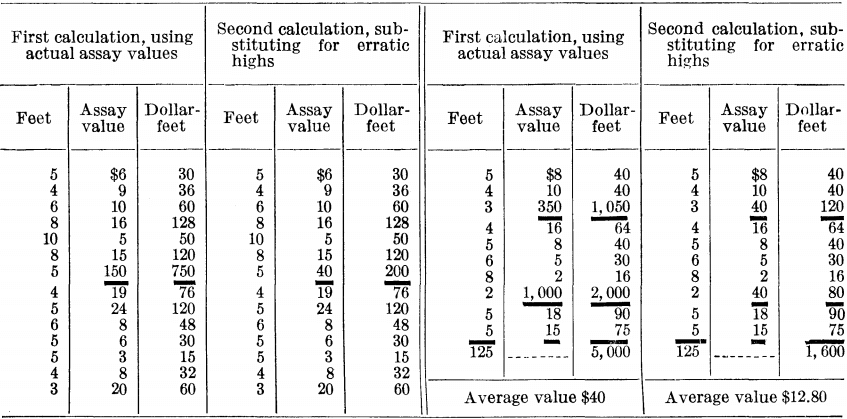## Computation of Tonnage and Grade of Ore by Blocks

Developed ore usually is calculated first for each of the different areas or blocks bounded by drifts, raises, winzes, and outlines of stoped areas. The volume of each block is its area times the average thickness of the ore in the block. The volume divided by the volume-per-ton factor gives the tons of ore in the block. Multiplying the tonnage in each block by its average assay value, adding the products, and dividing this sum by the sum of the tonnages gives the average assay value of all the blocks.

In computing average thickness and average grade, it is important to weight each sample or series of samples in accordance with the length, area, or volume it represents.

Even where samples are spaced at regular intervals, serious error may result from incorrect combining of assays if the grades of the individual samples or sections vary greatly. This is shown by the example given in figure 14, where a and b are drifts 300 feet long between raises c and d, 100 feet long connecting a and b. The figures show the lengths and values in dollars of samples that are cut at equal intervals around the block. The first figure is the length of the sample (equal to the thickness or width of the ore in this case).

If, in this example, equal weight is given to the products (width times grade) for all the samples, including the raise samples, the tonnage (using a factor of 12 cubic feet per ton) will be 17,812, the average grade \$7.98 per ton, and the total value of the ore in the block \$142,140. The average grade of the raise samples along c is seen to be higher than that of the other samples, and if given equal weight to the drift samples by simple averaging, their weight would be out of proportion to their actual influence and result in a value that is too high.

If, however, the block is divided as shown by the lines f f f f and g and the resulting triangular and trapezoidal blocks are computed separately, the end or raise samples will then be weighted more in accordance with their actual influence and a slightly greater tonnage, a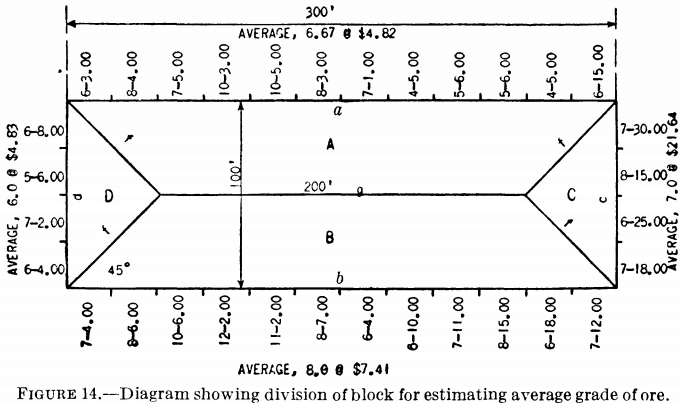lower average grade, and smaller total value will be obtained. The calculation is as follows: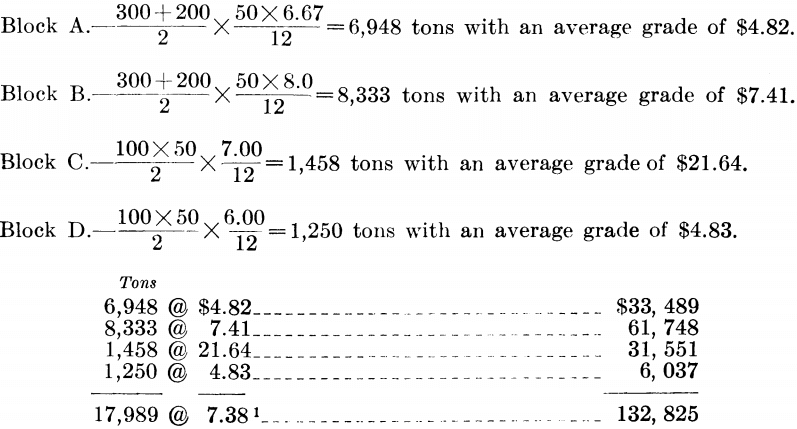## Revision of Computations

In the foregoing discussions the computations are mathematical, and the results obtained generally have to be modified to allow for mining and other factors peculiar to the particular deposit under investigation.

Thus, where random, irregular horses of waste are known to occur within the ore bodies, a deduction may have to be made from the calculated tonnage. This may be a percentage deduction based on past experience in mining the particular deposit or other similar deposits in the district, or it may be an engineering safety factor to provide for possible waste inclusions.

The computed figures may indeed be quite accurate as to tonnage and grade of ore actually in the deposit but may be quite different from the tonnage and grade of ore that actually can be recovered by mining. Thus, support of the surface may require that a considerable percentage of the total ore be left permanently in the form of pillars. In structurally weak ore bodies, in particular, it is seldom possible to prevent the loss of some ore in mining. This loss will depend on the irregularity of the ore margins and other characteristics of the deposits and may vary with the particular mining method employed, which may depend, in turn, on economic considerations, safety in working, and other factors. It is clear, therefore, that computed tonnages usually must be reduced by amounts based on these considerations to determine the amount of recoverable ore in the deposit. Reductions to take care of ore losses may or may not affect the resulting average grade of the recoverable ore. When pillars must be left, it is often possible to leave them in leaner ore, thus resulting in a higher than average grade for the ore that can be extracted. Conservative engineers, however, seldom would raise the computed grade on this account.

On the other hand, dilution from the walls of the deposit in stoping may considerably increase the total tonnage that will be mined, but this results definitely in reduction of the recoverable grade below the computed grade in proportion to the amount of dilution. The amount of dilution will vary with the nature of the deposit and the enclosing wall rocks and with the stoping method employed.

Frequently, hand sorting underground or on the surface is resorted to for the purpose of raising the grade of the ore shipped or sent to the mill. Some loss of ore almost invariably results, and although the grade of the product is raised, the tonnage to be treated is reduced, and, other things being equal, the total amount of metal recovered may be less. Actually, the total recovery in some instances may be more, since sorting may enable profitable mining of considerable tonnages of ore of too low-grade to be included as ore in the ore-reserve estimates.

Another problem often met is the recalculation of tonnages and grades where the vein width is less than the practicable stoping width. In vertical veins having firm walls it is sometimes possible to mine widths as narrow as 2 feet with little overbreak. More often, however, 36 to 40 inches is the narrowest average width that can be mined. If the vein is narrower than the practicable stoping width there must obviously be an overbreak in mining, and values of samples cut only over the vein width must be reduced to equivalent values over stoping width. Thus, assuming a vein averaging 2 feet in width and \$60 per ton and a minimum stoping width of 3 feet, the \$60 grade spread over 3 feet then becomes two-thirds of \$60, or \$40. That is, the grade of the material broken will be \$40, and if no sorting is done, the grade of ore shipped or sent to the mill will not exceed \$40 per ton.

All such factors must be considered in making the final estimates of recoverable ore and later in comuting probable future profits and in appraising the value of the property, a subject that will be discussed in a later chapter.

## Mine Development

The term “mine development” is employed to designate the operations involved in preparing a mine for ore extraction. These operations include tunneling, sinking, crosscutting, drifting, and raising.

In most mines, both exploration and development continue after ore extraction by stoping has begun and often nearly to the end of the life of the mine. Although exploration and development operations are similar, the emphasis is placed on ore finding in the former, whereas in the latter operations are directed mainly toward preparation for economical extraction and removal of the ore from the mine.

As previously pointed out, it may often be possible to drive exploratory openings in such positions and in such a manner that they may be utilized later for ore extraction. In other instances, however, openings driven with a view to expeditious and economical exploration may not be well-adapted to meet economical development and ore extraction.

Thus, an exploratory shaft may be too small for hoisting ore, men, materials, and supplies during the productive period and to provide room for pipes, electric-power cables, and ladderways. Exploratory drifts and crosscuts may be too small in cross section or too crooked for economical motor haulage, or they may be situated improperly with respect to stope centers. In other instances they may be in a lode that is structurally weak and in which, therefore, their maintenance cost would be so excessive that permanent haulageways must be driven in one or the other of the walls.

In mines situated in rugged mountainous districts and explored through shaft openings it may sometimes be desirable, for operating purposes, to drive a long adit to replace the shaft as the main extraction opening to eliminate high pumping and hoisting charges or to provide the most economical means of transporting the ore to an ore- dressing plant. Sometimes it is necessary or desirable to replace an exploratory inclined shaft by a vertical-operating shaft or to substitute one in the footwall of the lode for one in the lode itself.

Among the important factors to be considered in planning mine development for economical operation are the following:

1. Location of the main extraction opening.
2. Shaft vs. adit or tunnel development and inclined vs. vertical shafts.
3. Time required to prepare for production and anticipated maximum rate of production.
4. Level interval.
5. Level-development plans and their adaptation to the physical characteristics of the deposits and to the stoping method to be employed.
6. Life expectancy of the mine, total tonnage to be mined, and comparative costs of different development schemes.

Obviously, the type of ore deposit, its size, shape, and dip, distribution of ore shoots or separate ore bodies, strength of ore and wall rocks, and surface topography are conditions imposed by nature. It becomes necessary, therefore, to fit the development plan to these conditions, taking the utmost advantage of those that are favorable. It is apparent that the more complete the information obtained from previous exploration, the more intelligently can development be planned.